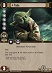Discussion about math, puzzles, games and fun.   Useful symbols: ÷ × ½ √ ∞ ≠ ≤ ≥ ≈ ⇒ ± ∈ Δ θ ∴ ∑ ∫  π  -¹ ² ³ °

You are not logged in.

## #1 2018-02-15 03:50:25

Monox D. I-Fly
MemberFrom: Indonesia
Registered: 2015-12-02
Posts: 1,383

A polynomial f(x) =

is divisible by (x - 3). The result of that polynomial f(x) divided by (x - 1) is ....
A.

B.

C.

D.

E.

I got a + 3 = -6 and so a = -9 and f(x) =

, but when I divided it with x - 1 I got
with the remainder 24. I'm quite sure that the question isn't wrong since it's in a national level exam, but where did I go wrong?

Actually I never watch Star Wars and not interested in it anyway, but I choose a Yoda card as my avatar in honor of our great friend bobbym who has passed away. May his adventurous soul rest in peace at heaven.

Offline

## #2 2018-02-15 07:13:11

bob bundyRegistered: 2010-06-20
Posts: 8,462

hi Monox D. I-Fly

I calculated a = -9 as well so I think that part is correct.

It'll be difficult to show a division on the forum so I'll try an alternative approach.  It amounts to the same thing but will allow me to proceed one step at a time.

2x^3-5x^2 -9x+18 is to be divided by (x-1) so the result will be a quadratic.  By inspection the x^2 term must be 2x^2 so that multiplying by x gives 2x^3

So progress so far : 2x^3-5x^2 -9x+18 = (x-1)(2x^2 +  ...) + .....

When -1 multiplies this quadratic term we get -1 times 2x^2 = -2x^2.  But the x^2 term is -5x^2 so we are 'short' by -3x^2.  That can only come from a linear term  multiplied by x ... implies the linear term must be -3x

So progress so far : 2x^3-5x^2 -9x+18 = (x-1)(2x^2 -3x + ...) + .....

-1 multiplied by -3x gives +3x.  We want -9x so -12x must come from somewhere.  It must come from a number multiplied by x.  So the number is -12

So progress so far : 2x^3-5x^2 -9x+18 = (x-1)(2x^2 -3x  -12) + .....

-1 multiplied by -12 = + 12.  We want + 18 so the remainder is +6

So:                        2x^3-5x^2 -9x+18 = (x-1)(2x^2 -3x  -12) + 6

Although I have not set this out as a division you should be able to check where you went wrong.

WHOOPS! That sin't any of the possible answers.  Just checking what I have done ..... Back soon.

LATER EDIT I've multiplied out my expression and get 2x^3 - 5x^2 - 9x + 18.  That's odd.  I'm going to use some heavier algebraic hammers to crack this puzzle.  Back soon.

EVEN LATER EDIT:  I've checked all my working by another method and find the same result.  Could these be the answers to another question entirely ?  Or could the original cubic be different ?

Bob

Before I started I used the remainder theorem to find the remainder.  I was expecting + 6 so that looks ok.

Last edited by bob bundy (2018-02-15 07:23:22)

Children are not defined by school ...........The Fonz
You cannot teach a man anything;  you can only help him find it within himself..........Galileo Galilei
Sometimes I deliberately make mistakes, just to test you!  …………….Bob BundyOffline

## #3 2018-02-16 17:21:49

Alg Num Theory
Member
Registered: 2017-11-24
Posts: 342
Website

so C would be the answer for division by (x+1).

Last edited by Alg Num Theory (2018-02-16 17:23:05)

Offline

## #4 2018-02-16 22:32:13

Monox D. I-Fly
MemberFrom: Indonesia
Registered: 2015-12-02
Posts: 1,383